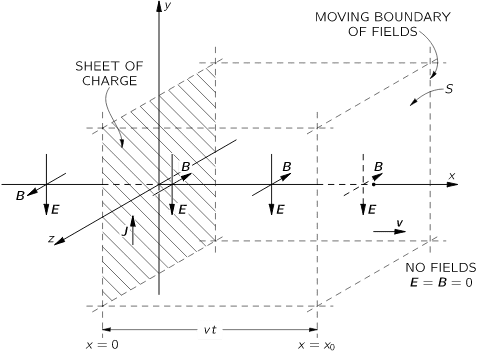# The phase of electric and magnetic forces in a photon.

merlyn
I'm curious if someone help me understand why the electric and magnetic forces are IN PHASE at right angles to one another?
Should they not be 90 degrees out of phase in order to conserve energy? I do understand they are in phase but why?

Thank you all for your time.

Merlyn.

Gold Member
2022 Award
Just solve the free Maxwell equations with the plane-wave ansatz
$$\vec{E}=\vec{E}_0 \exp(-\mathrm{i} \omega t + \mathrm{i} \vec{k} \cdot \vec{x}), \quad \vec{B}=\vec{B}_0 \exp(-\mathrm{i} \omega t + \mathrm{i} \vec{k} \cdot \vec{x}),$$
where it is understood that the physical fields are just the real parts of these complex-valued fields. The reason for using this trick is that it is easier to do the calculation with exponential rather than trigonometric functions.

Now (in Heaviside-Lorentz units) two free Maxwell equations, i.e., with ##\rho=0## and ##\vec{j}=0## read
$$\vec{\nabla} \cdot \vec{E}=\vec{\nabla} \cdot \vec{B}=0.$$
This implies
$$\vec{k} \cdot \vec{E}_0=\vec{k} \cdot \vec{B}_0=0,$$
i.e., both the electric and the magnetic fields are transverse waves.

Then you have
$$\vec{\nabla} \times \vec{E}+\frac{1}{c} \partial_t \vec{B}=0$$
and
$$\vec{\nabla} \times \vec{B}-\frac{1}{c} \partial_t \vec{E}=0.$$
Taking the curl of the first equation, using again ##\vec{\nabla} \cdot \vec{E}=0## and eliminating ##\vec{B}## with the 2nd equation leads to
$$\left (\frac{1}{c^2} \partial_t^2 -\Delta \right)\vec{E}=\Box \vec{E}=0.$$
In an analogous way you find
$$\Box{\vec{B}}=0.$$
Plugging in our plane-wave ansatz leads to
$$\frac{\omega^2}{c^2}-\vec{k}^2=0 \; \Rightarrow \; \omega = c |\vec{k}|=c k,$$
i.e., the usual dispersion relations for em. waves in a vacuum.

Now you also have
$$\vec{\nabla} \times \vec{E}=-\vec{E}_0 \times \vec{\nabla} \exp(\cdots) = -\mathrm{i} \vec{E}_0 \times \vec{k} \exp(\cdots) \stackrel{!}{=} -\frac{1}{c} \partial_t \vec{B}=\mathrm{i} \frac{\omega}{c} \vec{B}_0 \exp(\cdots).$$
Together with ##\omega=c/k## this leads to
$$\frac{\vec{k}}{k} \times \vec{E}_0=\vec{B}_0.$$
The other Maxwell equation doesn't lead to anything new but
$$\vec{E}_0=\vec{B}_0 \times \frac{\vec{k}}{k}.$$
This is equivalent to the previous equation, given that ##\vec{k} \cdot \vec{E}_0=\vec{k} \cdot \vec{B}_0=0##.

This implies that ##\vec{E}## and ##\vec{B}## are always in phase and perpendicular to each other.

To describe photons rather than classical fields, just read field operators instead of c-number valued fields.

Last edited:
•BvU
Mentor
with the plane-wave ansatz
It looks like your plane wave ansatz assumes the thing to be proven.

Gold Member
2022 Award
It looks like your plane wave ansatz assumes the thing to be proven.
No it doesn't, because ##\vec{E}_0## and ##\vec{B}_0## are arbitrary complex amplitudes. As my calculation shows, however
$$\vec{B}_0=\frac{\vec{k}}{k} \times \vec{E}_0,$$
and thus, since ##\vec{k}/k## is a real vector, there's no phase shift between ##\vec{B}_0## and ##\vec{E}_0##.

Gold Member
Should they not be 90 degrees out of phase in order to conserve energy?

In a standing wave the fields are 90 degrees out of phase.

You could write down traveling plane wave guesses for the electric and magnetic fields that were 90 out of phase and if you plug your guess into Maxwell's equations your guess won't satisfy Maxwell's equations? Maybe in the process of doing so you might come to an intuitive understanding why they are in phase in a traveling wave?

Feynman discusses in infinite plane of charge given sudden motion. It might help.

http://www.feynmanlectures.caltech.edu/II_18.html•vanhees71